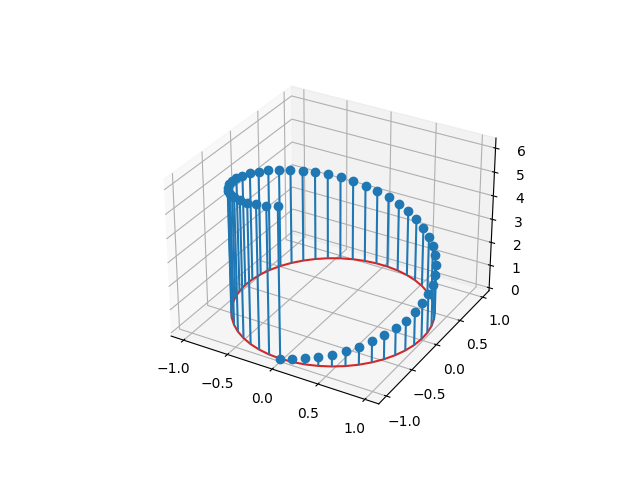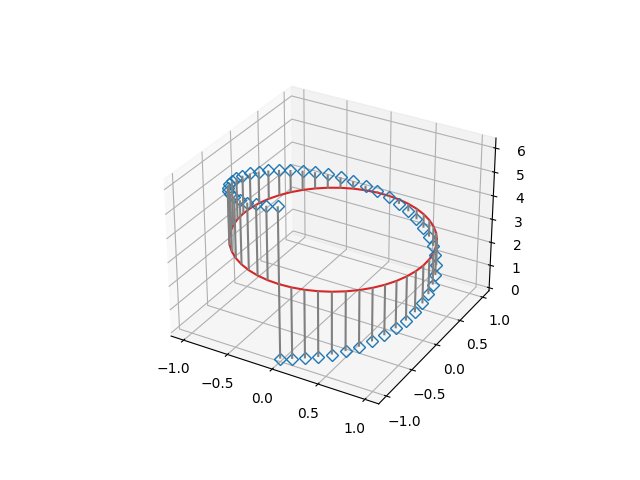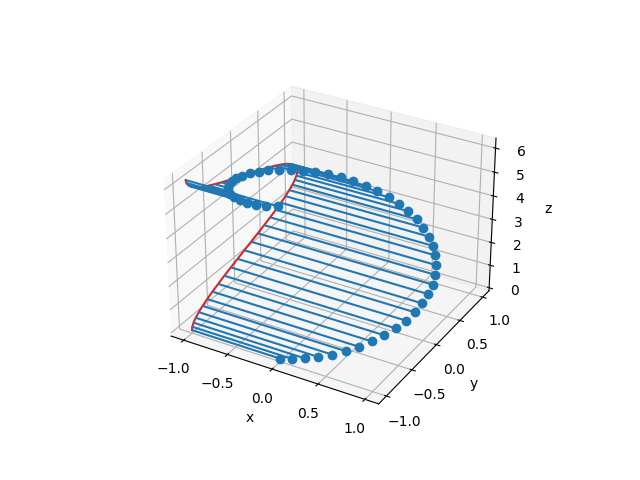# 3D stem¶

Demonstration of a stem plot in 3D, which plots vertical lines from a baseline to the z-coordinate and places a marker at the tip.

import matplotlib.pyplot as plt
import numpy as np

theta = np.linspace(0, 2*np.pi)
x = np.cos(theta - np.pi/2)
y = np.sin(theta - np.pi/2)
z = theta

fig, ax = plt.subplots(subplot_kw=dict(projection='3d'))
ax.stem(x, y, z)

plt.show()The position of the baseline can be adapted using bottom. The parameters linefmt, markerfmt, and basefmt control basic format properties of the plot. However, in contrast to plot not all properties are configurable via keyword arguments. For more advanced control adapt the line objects returned by stem3D.

fig, ax = plt.subplots(subplot_kw=dict(projection='3d'))
markerline, stemlines, baseline = ax.stem(
x, y, z, linefmt='grey', markerfmt='D', bottom=np.pi)
markerline.set_markerfacecolor('none')

plt.show()The orientation of the stems and baseline can be changed using orientation. This determines in which direction the stems are projected from the head points, towards the bottom baseline.

For examples, by setting orientation='x', the stems are projected along the x-direction, and the baseline is in the yz-plane.

fig, ax = plt.subplots(subplot_kw=dict(projection='3d'))
markerline, stemlines, baseline = ax.stem(x, y, z, bottom=-1, orientation='x')
ax.set(xlabel='x', ylabel='y', zlabel='z')

plt.show()Total running time of the script: ( 0 minutes 1.832 seconds)

Keywords: matplotlib code example, codex, python plot, pyplot Gallery generated by Sphinx-Gallery